## MP Board Class 7th Maths Solutions Chapter 11 Perimeter and Area Ex 11.3

Question 1.
Find the circumference of the circles with the following radius: (Take π = $$\frac{22}{7}$$)
(a) 14 cm
(b) 28 mm
(c) 21 cm
Solution:
(a) r = 14 cm
∴ Circumference = 2πr = 2 × $$\frac{22}{7}$$ × 14 =88 cm
(b) r = 28 mm
∴ Circumference = 2πr = 2 × $$\frac{22}{7}$$ × 28 = 176 mm
(c) r = 21 cm
∴ Circumference = 2πr = 2 × $$\frac{22}{7}$$ × 21 = 132 cm

Question 2.
Find the area of the following circles, given that: (Take π = $$\frac{22}{7}$$)
(b) diameter = 49 m
Solution:
(a) r = 14 mm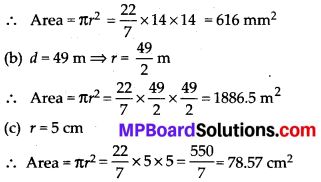Question 3.
If the circumference of a circular sheet is 154 m, find its radius. Also find the area of the sheet. (Take π = $$\frac{22}{7}$$)
Solution:
Circumference = 2πr = 154 m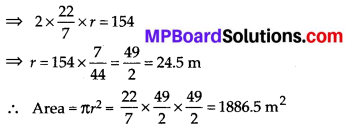Question 4.
A gardener wants to fence a circular garden of diameter 21 m. Find the length of the rope he needs to purchase, if he makes 2 rounds of fence. Also find the cost of the rope, if it costs ₹ 4 per meter. (Take π = $$\frac{22}{7}$$)
Solution:
Diameter (d) = 21 m 21
∴ Radius (r) = $$\frac{21}{2}$$m
Circumference = 2πr = 2 × $$\frac{22}{7} \times \frac{21}{2}$$ = 66 m
Length of rope required for fencing = 2 × 66 m = 132 m
Cost of 1 m rope = ₹ 4
Cost of 132 m rope = 4 × 132 = ₹ 528Question 5.
From a circular sheet of radius 4 cm, a circle of radius 3 cm is removed. Find the area of the remaining sheet. (Take π = 3.14)
Solution:
Outer radius of circular sheet (R) = 4 cm
Inner radius of circular sheet (r) = 3 cm
Remaining area = πR2 – πr2
= 3.14 × 4 × 4 – 3.14 × 3 × 3
= 50.24 – 28.26 = 21.98 cm2

Question 6.
Saima wants to put a lace on the edge of a circular table cover of diameter 1.5 m. Find the length of the lace required and also find its cost if one meter of the lace costs ₹ 15. (Take π = 3.14)
Solution:
The length of the lace required = circumference of circular table
Circumference = 2πr = 2 × 3.14 × $$\frac{d}{2}$$
= 2 × 3.14 × $$\frac{1.5}{2}$$ = 4.71 m
Cost of 1 m lace = ₹ 15
Cost of 4.71 m lace = 4.71 × 15 = ₹ 70.65

Question 7.
Find the perimeter of the adjoining figure, which is a semicircle including its diameter.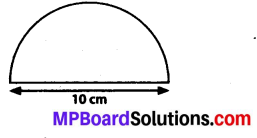Solution:
Diameter = 10 cm
Radius = $$\frac{10}{2}$$ = 5 cm
Circumference of semicircle = $$\frac{2 \pi r}{2}$$
= 2 × $$\frac{1}{2} \times \frac{22}{7}$$ × 5 = 15.71 cm
Total perimeter = Circumference of semicircle + Length of diameter
= 15.71 + 10 = 25.71 cm

Question 8.
Find the cost of polishing a circular table-top of diameter 1.6 m, if the rate of polishing is ₹ 15/m2. (Take π = 3.14)
Solution:
Diameter = 1.6 m
∴ Radius = $$\frac{1.6}{2}$$ = 0.8 m
Area = πr2 = 3.14 × 0.8 × 0.8 = 2.0096 m2
Cost for polishing 1 m2 area = ₹ 15
Cost for polishing 2.0096 m2 area
= 15 × 2.0096 = ₹ 30.14
Therefore, it will cost ₹ 30.14 for polishing circular table.Question 9.
Shazli took a wire of length 44 cm and bent it into the shape of a circle. Find the radius of that circle. Also find its area. If the same wire is bent into the shape of a square, what will be the length of each of its sides? Which figure encloses more area, the circle or the square? (Take π = $$\frac{22}{7}$$)
Solution:
If the wire is bent into a circle, then the length of wire = circumference of the circle
⇒ 2πr = 44 cm
⇒ 2 × $$\frac{22}{7}$$ × r = 44
⇒ r = 7 cm
Area = πr2= $$\frac{22}{7}$$ × 7 × 7 = 154 cm2
If the wire is bent into a square, then the length of the wire = perimeter of the square
⇒ 4 × side = 44cm ⇒ side = $$\frac{44}{4}$$ = 11 cm
Area of square = (11)2 = 121 cm2
As 154 > 121,
Therefore, circle encloses more area.

Question 10.
From a circular card sheet of radius 14 cm, two circles of radius 3.5 cm and a rectangle of length 3 cm and breadth 1 cm are removed (as shown in the following figure). Find the area of the remaining sheet. (Take π = $$\frac{22}{7}$$)Solution:
Area of bigger circle = $$\frac{22}{7}$$ × 14 × 14 = 616 cm2
Area of 2 small circles = 2 × πr2
= 2 × $$\frac{22}{7}$$ × 3.5 × 3.5 = 77 cm2
Area of rectangle = Length × Breadth = 3 × 1
= 3 cm2
Area of remaining sheet = Area of bigger circle – (Area of 2 small circles + Area of rectangle)
= 616 – (77 + 3) = 536 cm2

Question 11.
A circle of radius 2 cm is cut out from a square piece of an aluminium sheet of side 6 cm. What is the area of the left over aluminium sheet? (Take π = 3.14)
Solution:
Area of square-shaped sheet = (Side)2
= (6)2 = 36 cm2
Area of circle = 3.14 × 2 × 2= 12.56 cm2
Area of remaining sheet = Area of square sheet – area of circle
= 36 – 12.56 = 23.44 cm2

Question 12.
The circumference of a circle is 31.4 cm. Find the radius and the area of the circle? (Take π = 3.14)
Solution:
Let r be the radius of circle. Circumference = 2πr = 31.4 cm
⇒ 2 × 3.14 × r = 31.4 cm
⇒ r = 5 cm
Area = 3.14 × 5 × 5 = 78.50 cm2

Question 13.
A circular flower bed is surrounded by a path 4 m wide. The diameter of the flower bed is 66 m. What is the area of this path? (π = 3.14)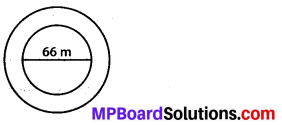Solution:
Radius of flower bed = $$\frac{66}{2}$$ = 33 m
Width of the path = 4 m
Radius of flower bed and path together = 33 + 4 = 37 m
Area of flower bed and path together
= 3.14 × 37 × 37 = 4298.66 m2
Area of flower bed = 3.14 × 33 × 33 = 3419.46 m2
Area of path = Area of flower bed and path together – Area of flower bed
= 4298.66 – 3419.46 = 879.20 m2

Question 14.
A circular flower garden has an area of 314 m2. A sprinkler at the centre of the garden can cover an area that has a radius of 12 m. Will the sprinkler water the entire garden? (Take π = 3.14)
Solution:
Area = πr2 = 314 m2
3.14 × r2 = 314 ⇒ r2 = 100 ⇒ r = 10 m
Yes, the sprinkler will water the whole garden.Question 15.
Find the circumference of the inner and the outer circles, shown in the adjoining figure? (Take π = 3.14)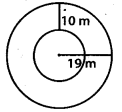Solution:
Radius of outer circle = 19 m
Circumference = 2πr =2 × 3.14 × 19 = 119.32 m
Radius of inner circle = 19 – 10 = 9 m
Circumference = 2πr = 2 × 3.14 × 9 = 56.52 m

Question 16.
How many times a wheel of radius 28 cm must rotate to go 352 m? (Take π = $$\frac{22}{7}$$)
Solution:
r = 78 cm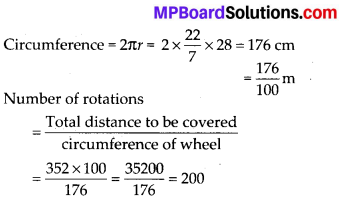Therefore, it will rotate 200 times.

Question 17.
The minute hand of a circular clock is 15 cm long. How far does the tip of the minute hand move in 1 hour? (Take π = 3.14)
Solution:
Distance travelled by the tip of minute hand = Circumference of the clock
= 2πr = 2 × 3.14 × 15 = 94.2 cm